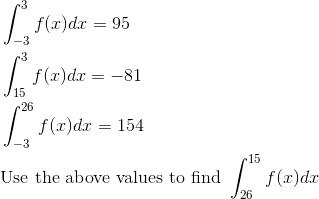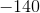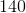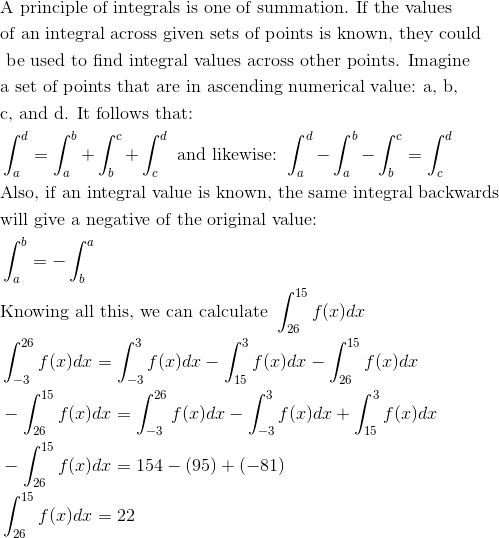# AP Calculus AB : Basic properties of definite integrals (additivity and linearity)

## Example Questions

### Example Question #11 : Basic Properties Of Definite Integrals (Additivity And Linearity)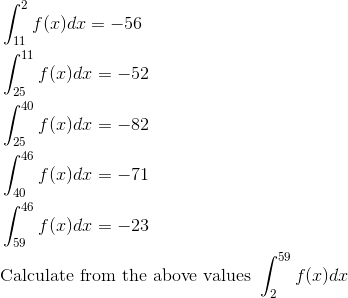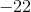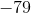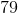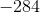Explanation: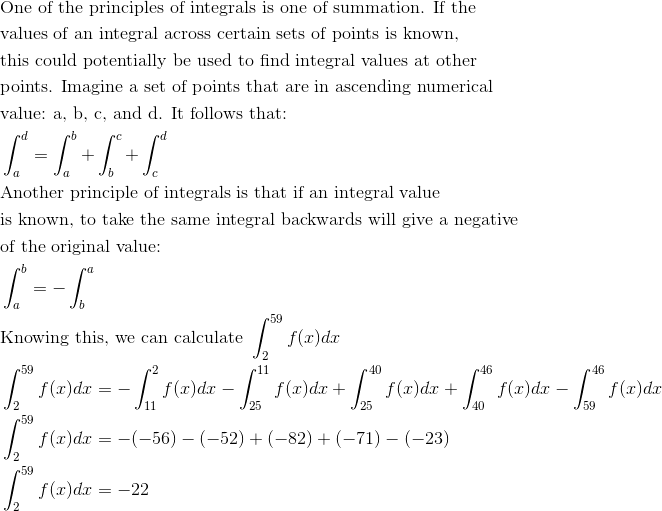### Example Question #12 : Basic Properties Of Definite Integrals (Additivity And Linearity)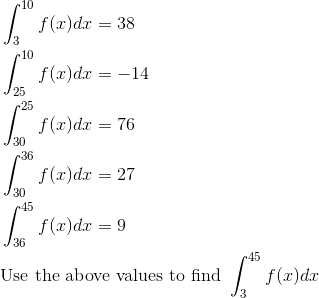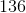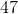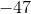Explanation: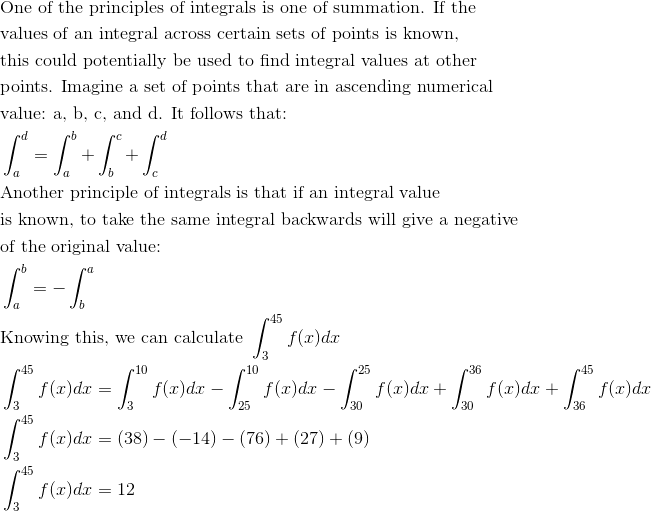### Example Question #13 : Basic Properties Of Definite Integrals (Additivity And Linearity)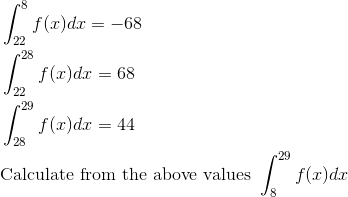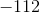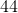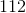Explanation: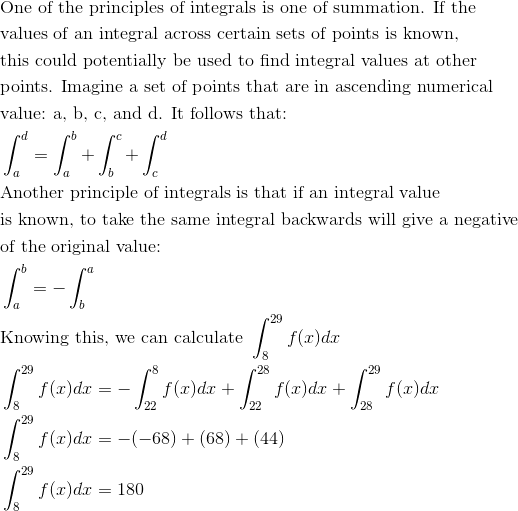### Example Question #14 : Basic Properties Of Definite Integrals (Additivity And Linearity)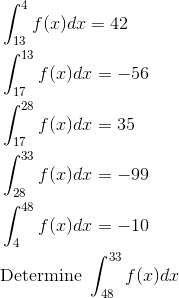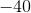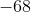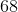Explanation: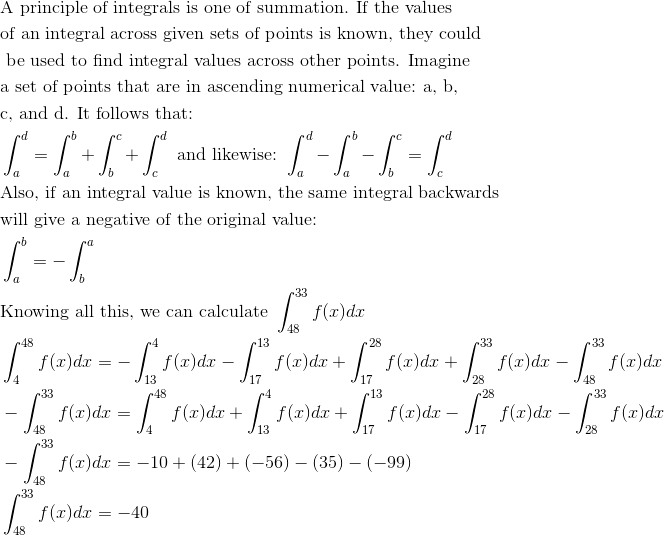### Example Question #151 : Integrals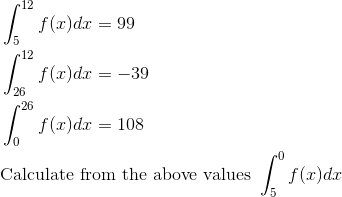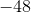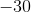Explanation: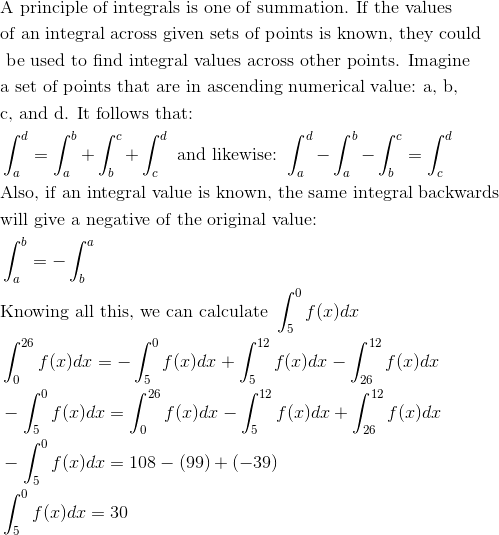### Example Question #15 : Basic Properties Of Definite Integrals (Additivity And Linearity)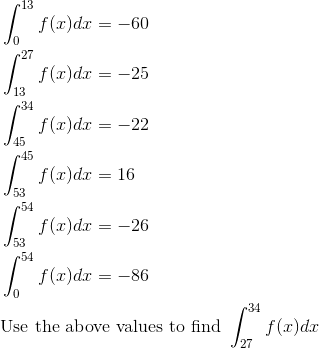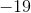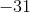Explanation: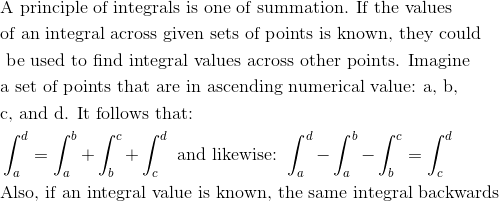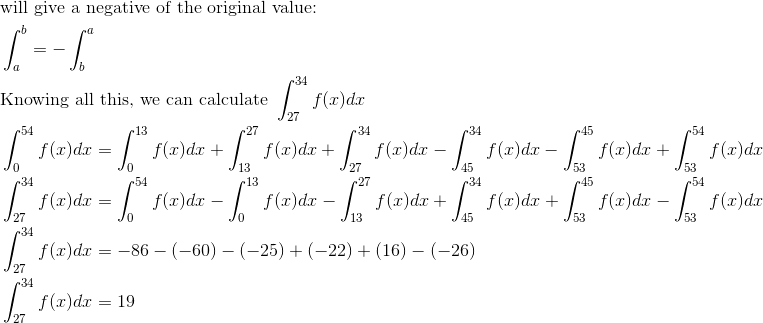### Example Question #16 : Basic Properties Of Definite Integrals (Additivity And Linearity)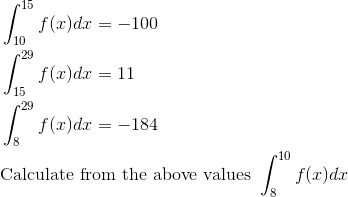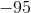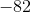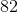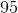Explanation: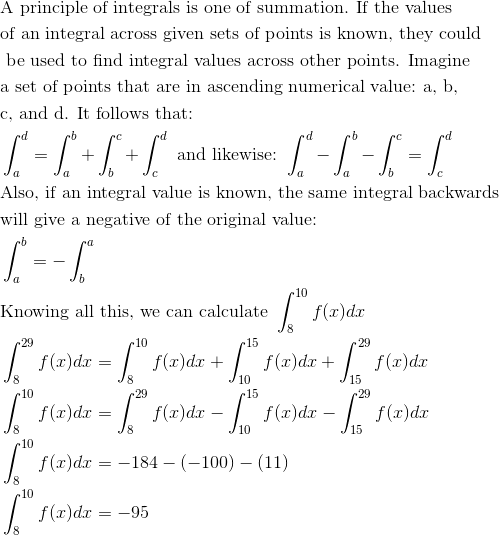### Example Question #17 : Basic Properties Of Definite Integrals (Additivity And Linearity)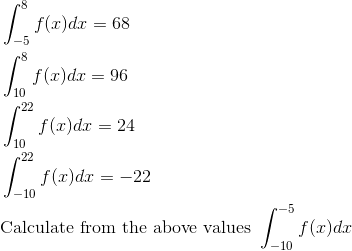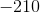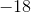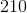Explanation: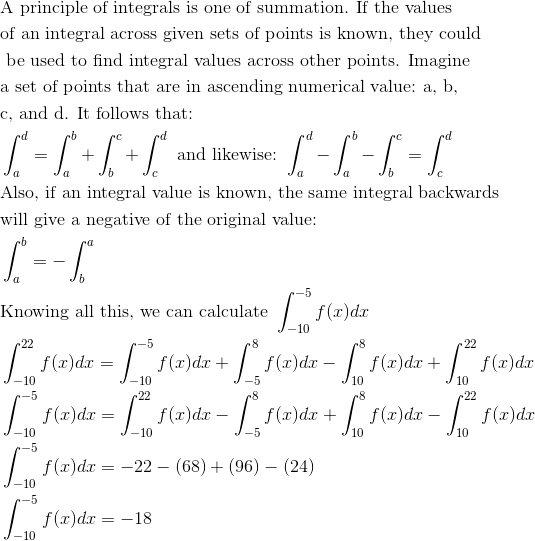### Example Question #18 : Basic Properties Of Definite Integrals (Additivity And Linearity)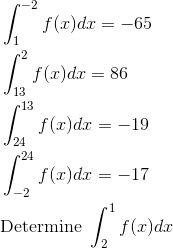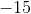Explanation: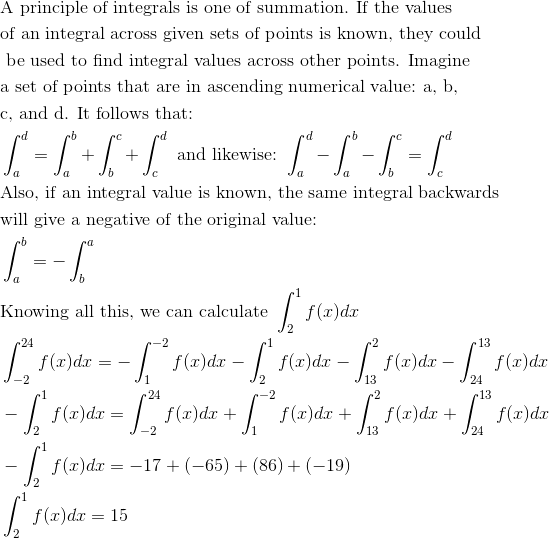### Example Question #19 : Basic Properties Of Definite Integrals (Additivity And Linearity)